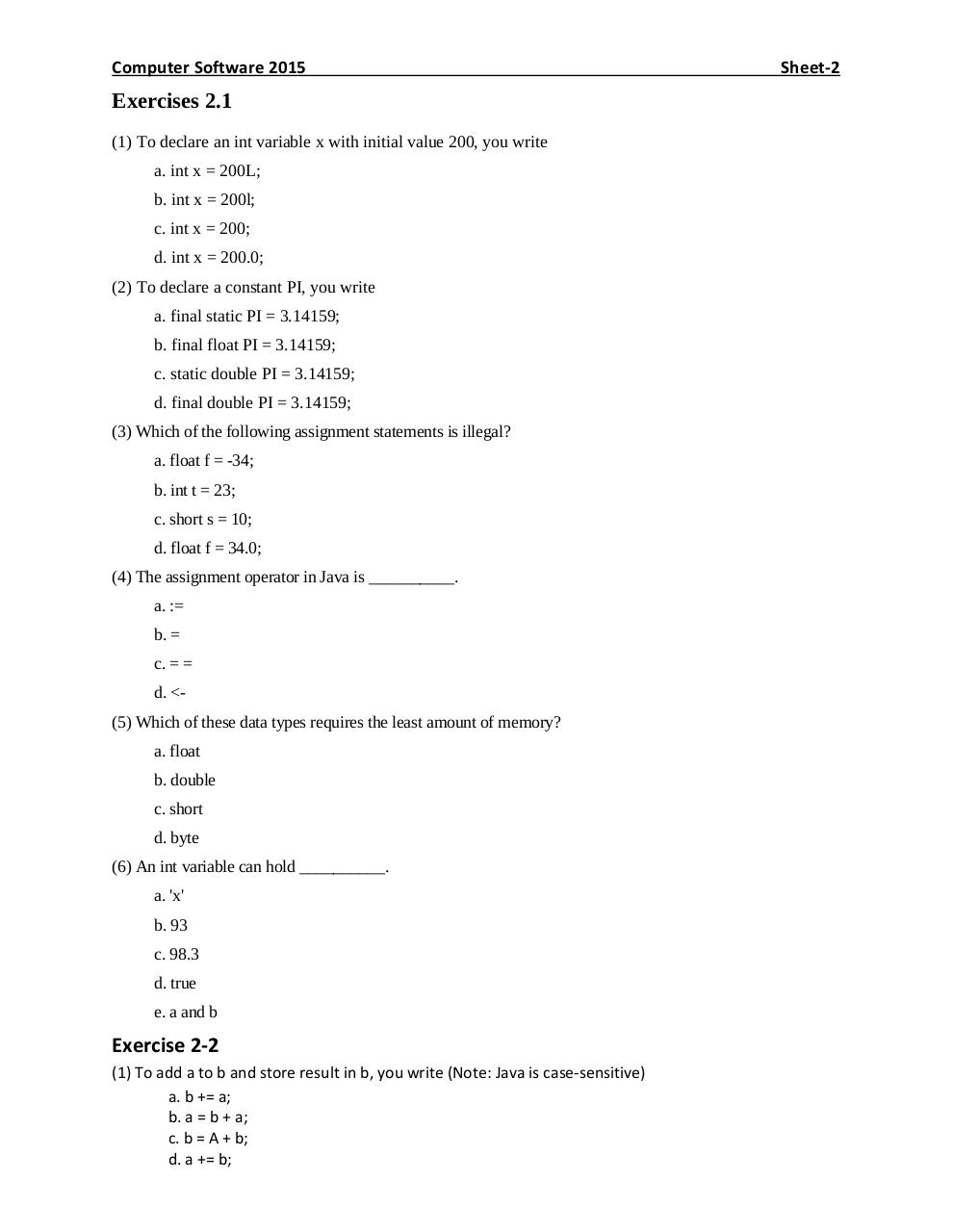# Sheet 2 2015.pdfPage 1 2 3 4 5 6 7

#### Text preview

Computer Software 2015

Exercises 2.1
(1) To declare an int variable x with initial value 200, you write
a. int x = 200L;
b. int x = 200l;
c. int x = 200;
d. int x = 200.0;
(2) To declare a constant PI, you write
a. final static PI = 3.14159;
b. final float PI = 3.14159;
c. static double PI = 3.14159;
d. final double PI = 3.14159;
(3) Which of the following assignment statements is illegal?
a. float f = -34;
b. int t = 23;
c. short s = 10;
d. float f = 34.0;
(4) The assignment operator in Java is __________.
a. :=
b. =
c. = =
d. &lt;(5) Which of these data types requires the least amount of memory?
a. float
b. double
c. short
d. byte
(6) An int variable can hold __________.
a. 'x'
b. 93
c. 98.3
d. true
e. a and b

Exercise 2-2
(1) To add a to b and store result in b, you write (Note: Java is case-sensitive)
a. b += a;
b. a = b + a;
c. b = A + b;
d. a += b;

Sheet-2